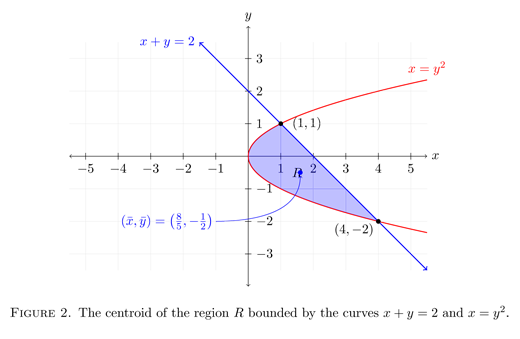# Jamie's question from Yahoo! Answers regarding centroids

Staff member

#### Chris L T521

##### Well-known member
Staff member
Hi Jamie,

The region that we're supposed to find the centroid for can be found in the figure below.Assuming that you know multivariable calculus, one defines the centroid as $(\bar{x},\bar{y})$ where
$\bar{x}= \frac{\displaystyle\iint\limits_R x\,dA}{\displaystyle\iint\limits_R \,dA}=\frac{1}{\text{Area of R}}\iint\limits_R x\,dA\qquad\text{ and }\qquad \bar{y}= \frac{\displaystyle\iint\limits_R y\,dA}{\displaystyle\iint\limits_R \,dA}=\frac{1}{\text{Area of R}}\iint\limits_R y\,dA$
Due to the region $R$ we have, it would be best to treat it as a type II region and treat the bounding functions as functions of the form $x=f(y)$ (if we treated the region as type I, then we'd have more than one integral to work with for each of $\bar{x}$ and $\bar{y}$). With that said, the two bounding functions are $x=2-y$ and $x=y^2$.

Thus, we see that
\begin{aligned} \iint\limits_R \,dA &= \int_{-2}^1\int_{y^2}^{2-y}\,dx\,dy \\ &= \int_{-2}^1 2-y-y^2\,dy \\ &= \left.\left[ 2y-\frac{1}{2}y^2-\frac{1}{3}y^3\right]\right|_{-2}^1\\ &= \left(2-\frac{1}{2}-\frac{1}{3}\right) - \left(-4 -2 + \frac{8}{3}\right)\\ &= \frac{9}{2}\end{aligned}

\begin{aligned} \iint\limits_R x\,dA &= \int_{-2}^1\int_{y^2}^{2-y} x\,dx\,dy\\ &= \frac{1}{2}\int_{-2}^1 \left.\left[ x^2\right]\right|_{y^2}^{2-y}\,dy\\ &= \frac{1}{2}\int_{-2}^1 4-4y+y^2-y^4\,dy \\ &= \frac{1}{2}\left.\left[ 4y - 2y^2+\frac{1}{3}y^3- \frac{1}{5}y^5\right]\right|_{-2}^1\\ &= \frac{1}{2}\left[\left( 4-2+\frac{1}{3}-\frac{1}{5}\right) - \left( -8-8-\frac{8}{3} +\frac{32}{5}\right)\right]\\ &= \frac{36}{5}\end{aligned}

\begin{aligned} \iint\limits_R y\,dA &= \int_{-2}^1\int_{y^2}^{2-y} y\,dx\,dy\\ &= \int_{-2}^1 y(2-y-y^2)\,dy\\ &= \int_{-2}^1 2y-y^2-y^3\,dy \\ &= \left.\left[y^2-\frac{1}{3}y^3-\frac{1}{4}y^4\right]\right|_{-2}^1\\ &= \left[\left(1-\frac{1}{3}-\frac{1}{4}\right) - \left(4+\frac{8}{3} - 4\right)\right]\\ &= -\frac{9}{4}\end{aligned}

Therefore,

$\bar{x}= \frac{\displaystyle\iint\limits_R x\,dA}{\displaystyle\iint\limits_R \,dA}= \frac{\dfrac{36}{5}}{\dfrac{9}{2}} = \frac{8}{5}$

and

$\bar{y}= \frac{\displaystyle\iint\limits_R y\,dA}{\displaystyle\iint\limits_R \,dA}= \frac{-\dfrac{9}{4}}{\dfrac{9}{2}} = -\frac{1}{2}$

Thus, the centroid of this region is $(\bar{x},\bar{y})=\left(\dfrac{8}{5},-\dfrac{1}{2}\right)$ (as seen in the figure below).#### Chris L T521

##### Well-known member
Staff member
For those of you who are interested, here's the TikZ codes for the two figures (in two separate posts because apparently I've exceeded the character limit for a single post).Code:
[COLOR=#0000CC]\begin[/COLOR][COLOR=#000000]{figure}[![/COLOR][COLOR=#000000]ht[/COLOR][COLOR=#000000]][/COLOR]
[COLOR=#800000]\centering[/COLOR]
[COLOR=#0000cc]\begin[/COLOR][COLOR=#000000]{tikzpicture}[/COLOR][COLOR=#9a4d00][scale=[/COLOR][COLOR=#660066].95[/COLOR][COLOR=#9a4d00]][/COLOR]
[COLOR=#006699]   \draw[/COLOR][COLOR=#9a4d00][very thin,color=gray![/COLOR][COLOR=#660066]35[/COLOR][COLOR=#9a4d00]] (-[/COLOR][COLOR=#660066]5.5[/COLOR][COLOR=#9a4d00],-[/COLOR][COLOR=#660066]3.5[/COLOR][COLOR=#9a4d00]) grid ([/COLOR][COLOR=#660066]5.5[/COLOR][COLOR=#9a4d00],[/COLOR][COLOR=#660066]3.5[/COLOR][COLOR=#9a4d00]);[/COLOR]
[COLOR=#006699]   \draw[/COLOR][COLOR=#9a4d00][<->] (-[/COLOR][COLOR=#660066]5.5[/COLOR][COLOR=#9a4d00],[/COLOR][COLOR=#660066]0[/COLOR][COLOR=#9a4d00]) -- ([/COLOR][COLOR=#660066]5.5[/COLOR][COLOR=#9a4d00],[/COLOR][COLOR=#660066]0[/COLOR][COLOR=#9a4d00]) node[right]{[/COLOR][COLOR=#008000]$x$[/COLOR][COLOR=#9a4d00]};[/COLOR]
[COLOR=#006699]   \draw[/COLOR][COLOR=#9a4d00][<->] ([/COLOR][COLOR=#660066]0[/COLOR][COLOR=#9a4d00],-[/COLOR][COLOR=#660066]4[/COLOR][COLOR=#9a4d00]) -- ([/COLOR][COLOR=#660066]0[/COLOR][COLOR=#9a4d00],[/COLOR][COLOR=#660066]4[/COLOR][COLOR=#9a4d00]) node[above]{[/COLOR][COLOR=#008000]$y$[/COLOR][COLOR=#9a4d00]};[/COLOR]
[COLOR=#006699]   \foreach[/COLOR][COLOR=#006699]\x[/COLOR][COLOR=#9a4d00] in {-[/COLOR][COLOR=#660066]5[/COLOR][COLOR=#9a4d00],-[/COLOR][COLOR=#660066]4[/COLOR][COLOR=#9a4d00],-[/COLOR][COLOR=#660066]3[/COLOR][COLOR=#9a4d00],-[/COLOR][COLOR=#660066]2[/COLOR][COLOR=#9a4d00],-[/COLOR][COLOR=#660066]1[/COLOR][COLOR=#9a4d00],[/COLOR][COLOR=#660066]1[/COLOR][COLOR=#9a4d00],[/COLOR][COLOR=#660066]2[/COLOR][COLOR=#9a4d00],[/COLOR][COLOR=#660066]3[/COLOR][COLOR=#9a4d00],[/COLOR][COLOR=#660066]4[/COLOR][COLOR=#9a4d00],[/COLOR][COLOR=#660066]5[/COLOR][COLOR=#9a4d00]}{[/COLOR]
[COLOR=#006699]   \draw[/COLOR][COLOR=#9a4d00] ([/COLOR][COLOR=#006699]\x[/COLOR][COLOR=#9a4d00],[/COLOR][COLOR=#660066].1[/COLOR][COLOR=#9a4d00]) -- ([/COLOR][COLOR=#006699]\x[/COLOR][COLOR=#9a4d00],-[/COLOR][COLOR=#660066].1[/COLOR][COLOR=#9a4d00]) node[below]{[/COLOR][COLOR=#008000]$\x$[/COLOR][COLOR=#9a4d00]};[/COLOR]
[COLOR=#9a4d00]      }[/COLOR]
[COLOR=#006699]   \foreach[/COLOR][COLOR=#006699]\x[/COLOR][COLOR=#9a4d00] in {-[/COLOR][COLOR=#660066]3[/COLOR][COLOR=#9a4d00],-[/COLOR][COLOR=#660066]2[/COLOR][COLOR=#9a4d00],-[/COLOR][COLOR=#660066]1[/COLOR][COLOR=#9a4d00],[/COLOR][COLOR=#660066]1[/COLOR][COLOR=#9a4d00],[/COLOR][COLOR=#660066]2[/COLOR][COLOR=#9a4d00],[/COLOR][COLOR=#660066]3[/COLOR][COLOR=#9a4d00]}{[/COLOR]
[COLOR=#006699]   \draw[/COLOR][COLOR=#9a4d00] (-[/COLOR][COLOR=#660066].1[/COLOR][COLOR=#9a4d00],[/COLOR][COLOR=#006699]\x[/COLOR][COLOR=#9a4d00]) -- ([/COLOR][COLOR=#660066].1[/COLOR][COLOR=#9a4d00],[/COLOR][COLOR=#006699]\x[/COLOR][COLOR=#9a4d00]) node[right]{[/COLOR][COLOR=#008000]$\x$[/COLOR][COLOR=#9a4d00]};[/COLOR]
[COLOR=#9a4d00]     }[/COLOR]
[COLOR=#006699]   \draw[/COLOR][COLOR=#9a4d00][<->,blue,thick]([/COLOR][COLOR=#660066]5.5[/COLOR][COLOR=#9a4d00],-[/COLOR][COLOR=#660066]3.5[/COLOR][COLOR=#9a4d00]) -- (-[/COLOR][COLOR=#660066]1.5[/COLOR][COLOR=#9a4d00],[/COLOR][COLOR=#660066]3.5[/COLOR][COLOR=#9a4d00]) node[above,left]{[/COLOR][COLOR=#008000]$x+y=2$[/COLOR][COLOR=#9a4d00]};[/COLOR]
[COLOR=#006699]   \fill[/COLOR][COLOR=#9a4d00][color=blue,opacity=[/COLOR][COLOR=#660066].25[/COLOR][COLOR=#9a4d00]] ([/COLOR][COLOR=#660066]1[/COLOR][COLOR=#9a4d00],[/COLOR][COLOR=#660066]1[/COLOR][COLOR=#9a4d00]) -- ([/COLOR][COLOR=#660066]4[/COLOR][COLOR=#9a4d00],-[/COLOR][COLOR=#660066]2[/COLOR][COLOR=#9a4d00]) -- plot[domain=[/COLOR][COLOR=#660066]0[/COLOR][COLOR=#9a4d00]:[/COLOR][COLOR=#660066]4[/COLOR][COLOR=#9a4d00],smooth,samples=[/COLOR][COLOR=#660066]1500[/COLOR][COLOR=#9a4d00]]([/COLOR][COLOR=#006699]\x[/COLOR][COLOR=#9a4d00],{-sqrt([/COLOR][COLOR=#006699]\x[/COLOR][COLOR=#9a4d00])})
-- plot[domain=[/COLOR][COLOR=#660066]0[/COLOR][COLOR=#9a4d00]:[/COLOR][COLOR=#660066]1[/COLOR][COLOR=#9a4d00],smooth,samples=[/COLOR][COLOR=#660066]1500[/COLOR][COLOR=#9a4d00]]([/COLOR][COLOR=#006699]\x[/COLOR][COLOR=#9a4d00],{sqrt([/COLOR][COLOR=#006699]\x[/COLOR][COLOR=#9a4d00])}) --  cycle;[/COLOR]
[COLOR=#006699]   \draw[/COLOR][COLOR=#9a4d00] ([/COLOR][COLOR=#660066]1.5[/COLOR][COLOR=#9a4d00],-[/COLOR][COLOR=#660066]0.5[/COLOR][COLOR=#9a4d00]) node{[/COLOR][COLOR=#008000]$R$[/COLOR][COLOR=#9a4d00]};[/COLOR]
[COLOR=#006699]   \draw[/COLOR][COLOR=#9a4d00][red,thick] plot[domain=[/COLOR][COLOR=#660066]0[/COLOR][COLOR=#9a4d00]:[/COLOR][COLOR=#660066]5.5[/COLOR][COLOR=#9a4d00],smooth,samples=[/COLOR][COLOR=#660066]1500[/COLOR][COLOR=#9a4d00]] ([/COLOR][COLOR=#006699]\x[/COLOR][COLOR=#9a4d00], {-sqrt([/COLOR][COLOR=#006699]\x[/COLOR][COLOR=#9a4d00])});[/COLOR]
[COLOR=#006699]   \draw[/COLOR][COLOR=#9a4d00][red,thick] plot[domain=[/COLOR][COLOR=#660066]0[/COLOR][COLOR=#9a4d00]:[/COLOR][COLOR=#660066]5.5[/COLOR][COLOR=#9a4d00],smooth,samples=[/COLOR][COLOR=#660066]1500[/COLOR][COLOR=#9a4d00]] ([/COLOR][COLOR=#006699]\x[/COLOR][COLOR=#9a4d00], {sqrt([/COLOR][COLOR=#006699]\x[/COLOR][COLOR=#9a4d00])}) node[above]{[/COLOR][COLOR=#008000]$x=y^2$[/COLOR][COLOR=#9a4d00]};[/COLOR]
[COLOR=#006699]   \fill[/COLOR][COLOR=#9a4d00] ([/COLOR][COLOR=#660066]1[/COLOR][COLOR=#9a4d00],[/COLOR][COLOR=#660066]1[/COLOR][COLOR=#9a4d00]) circle ([/COLOR][COLOR=#660066]2[/COLOR][COLOR=#9a4d00]pt) node[right=[/COLOR][COLOR=#660066].2[/COLOR][COLOR=#9a4d00]cm]{[/COLOR][COLOR=#008000]$(1,1)$[/COLOR][COLOR=#9a4d00]};[/COLOR]
[COLOR=#006699]   \fill[/COLOR][COLOR=#9a4d00] ([/COLOR][COLOR=#660066]4[/COLOR][COLOR=#9a4d00],-[/COLOR][COLOR=#660066]2[/COLOR][COLOR=#9a4d00]) circle ([/COLOR][COLOR=#660066]2[/COLOR][COLOR=#9a4d00]pt) node[below=[/COLOR][COLOR=#660066].25[/COLOR][COLOR=#9a4d00]cm,left]{[/COLOR][COLOR=#008000]$(4,-2)$[/COLOR][COLOR=#9a4d00]};[/COLOR]
[COLOR=#0000cc]\end[/COLOR][COLOR=#000000]{tikzpicture}[/COLOR]
[COLOR=#800000]\caption[/COLOR][COLOR=#000000]{The region [/COLOR][COLOR=#008000]$R$[/COLOR][COLOR=#000000] bounded by the curves [/COLOR][COLOR=#008000]$x+y=2$[/COLOR][COLOR=#000000] and [/COLOR][COLOR=#008000]$x=y^2$[/COLOR][COLOR=#000000].}
[/COLOR][COLOR=#0000CC]\end[/COLOR][COLOR=#000000]{figure}[/COLOR]

#### Chris L T521

##### Well-known member
Staff memberCode:
[COLOR=#0000CC]\begin[/COLOR][COLOR=#000000]{figure}[![/COLOR][COLOR=#000000]ht[/COLOR][COLOR=#000000]][/COLOR]
[COLOR=#800000]\centering[/COLOR]
[COLOR=#0000cc]\begin[/COLOR][COLOR=#000000]{tikzpicture}[/COLOR][COLOR=#9a4d00][scale=[/COLOR][COLOR=#660066].95[/COLOR][COLOR=#9a4d00]][/COLOR]
[COLOR=#006699]   \draw[/COLOR][COLOR=#9a4d00][very thin,color=gray![/COLOR][COLOR=#660066]35[/COLOR][COLOR=#9a4d00]] (-[/COLOR][COLOR=#660066]5.5[/COLOR][COLOR=#9a4d00],-[/COLOR][COLOR=#660066]3.5[/COLOR][COLOR=#9a4d00]) grid ([/COLOR][COLOR=#660066]5.5[/COLOR][COLOR=#9a4d00],[/COLOR][COLOR=#660066]3.5[/COLOR][COLOR=#9a4d00]);[/COLOR]
[COLOR=#006699]   \draw[/COLOR][COLOR=#9a4d00][<->] (-[/COLOR][COLOR=#660066]5.5[/COLOR][COLOR=#9a4d00],[/COLOR][COLOR=#660066]0[/COLOR][COLOR=#9a4d00]) -- ([/COLOR][COLOR=#660066]5.5[/COLOR][COLOR=#9a4d00],[/COLOR][COLOR=#660066]0[/COLOR][COLOR=#9a4d00]) node[right]{[/COLOR][COLOR=#008000]$x$[/COLOR][COLOR=#9a4d00]};[/COLOR]
[COLOR=#006699]   \draw[/COLOR][COLOR=#9a4d00][<->] ([/COLOR][COLOR=#660066]0[/COLOR][COLOR=#9a4d00],-[/COLOR][COLOR=#660066]4[/COLOR][COLOR=#9a4d00]) -- ([/COLOR][COLOR=#660066]0[/COLOR][COLOR=#9a4d00],[/COLOR][COLOR=#660066]4[/COLOR][COLOR=#9a4d00]) node[above]{[/COLOR][COLOR=#008000]$y$[/COLOR][COLOR=#9a4d00]};[/COLOR]
[COLOR=#006699]   \foreach[/COLOR][COLOR=#006699]\x[/COLOR][COLOR=#9a4d00] in {-[/COLOR][COLOR=#660066]5[/COLOR][COLOR=#9a4d00],-[/COLOR][COLOR=#660066]4[/COLOR][COLOR=#9a4d00],-[/COLOR][COLOR=#660066]3[/COLOR][COLOR=#9a4d00],-[/COLOR][COLOR=#660066]2[/COLOR][COLOR=#9a4d00],-[/COLOR][COLOR=#660066]1[/COLOR][COLOR=#9a4d00],[/COLOR][COLOR=#660066]1[/COLOR][COLOR=#9a4d00],[/COLOR][COLOR=#660066]2[/COLOR][COLOR=#9a4d00],[/COLOR][COLOR=#660066]3[/COLOR][COLOR=#9a4d00],[/COLOR][COLOR=#660066]4[/COLOR][COLOR=#9a4d00],[/COLOR][COLOR=#660066]5[/COLOR][COLOR=#9a4d00]}{[/COLOR]
[COLOR=#006699]   \draw[/COLOR][COLOR=#9a4d00] ([/COLOR][COLOR=#006699]\x[/COLOR][COLOR=#9a4d00],[/COLOR][COLOR=#660066].1[/COLOR][COLOR=#9a4d00]) -- ([/COLOR][COLOR=#006699]\x[/COLOR][COLOR=#9a4d00],-[/COLOR][COLOR=#660066].1[/COLOR][COLOR=#9a4d00]) node[below]{[/COLOR][COLOR=#008000]$\x$[/COLOR][COLOR=#9a4d00]};[/COLOR]
[COLOR=#9a4d00]     }[/COLOR]
[COLOR=#006699]   \foreach[/COLOR][COLOR=#006699]\x[/COLOR][COLOR=#9a4d00] in {-[/COLOR][COLOR=#660066]3[/COLOR][COLOR=#9a4d00],-[/COLOR][COLOR=#660066]2[/COLOR][COLOR=#9a4d00],-[/COLOR][COLOR=#660066]1[/COLOR][COLOR=#9a4d00],[/COLOR][COLOR=#660066]1[/COLOR][COLOR=#9a4d00],[/COLOR][COLOR=#660066]2[/COLOR][COLOR=#9a4d00],[/COLOR][COLOR=#660066]3[/COLOR][COLOR=#9a4d00]}{[/COLOR]
[COLOR=#006699]   \draw[/COLOR][COLOR=#9a4d00] (-[/COLOR][COLOR=#660066].1[/COLOR][COLOR=#9a4d00],[/COLOR][COLOR=#006699]\x[/COLOR][COLOR=#9a4d00]) -- ([/COLOR][COLOR=#660066].1[/COLOR][COLOR=#9a4d00],[/COLOR][COLOR=#006699]\x[/COLOR][COLOR=#9a4d00]) node[right]{[/COLOR][COLOR=#008000]$\x$[/COLOR][COLOR=#9a4d00]};[/COLOR]
[COLOR=#9a4d00]     }[/COLOR]
[COLOR=#006699]   \draw[/COLOR][COLOR=#9a4d00][<->,blue,thick]([/COLOR][COLOR=#660066]5.5[/COLOR][COLOR=#9a4d00],-[/COLOR][COLOR=#660066]3.5[/COLOR][COLOR=#9a4d00]) -- (-[/COLOR][COLOR=#660066]1.5[/COLOR][COLOR=#9a4d00],[/COLOR][COLOR=#660066]3.5[/COLOR][COLOR=#9a4d00]) node[above,left]{[/COLOR][COLOR=#008000]$x+y=2$[/COLOR][COLOR=#9a4d00]};[/COLOR]
[COLOR=#006699]   \fill[/COLOR][COLOR=#9a4d00][color=blue,opacity=[/COLOR][COLOR=#660066].25[/COLOR][COLOR=#9a4d00]] ([/COLOR][COLOR=#660066]1[/COLOR][COLOR=#9a4d00],[/COLOR][COLOR=#660066]1[/COLOR][COLOR=#9a4d00]) -- ([/COLOR][COLOR=#660066]4[/COLOR][COLOR=#9a4d00],-[/COLOR][COLOR=#660066]2[/COLOR][COLOR=#9a4d00]) -- plot[domain=[/COLOR][COLOR=#660066]0[/COLOR][COLOR=#9a4d00]:[/COLOR][COLOR=#660066]4[/COLOR][COLOR=#9a4d00],smooth,samples=[/COLOR][COLOR=#660066]1500[/COLOR][COLOR=#9a4d00]]([/COLOR][COLOR=#006699]\x[/COLOR][COLOR=#9a4d00],{-sqrt([/COLOR][COLOR=#006699]\x[/COLOR][COLOR=#9a4d00])})
-- plot[domain=[/COLOR][COLOR=#660066]0[/COLOR][COLOR=#9a4d00]:[/COLOR][COLOR=#660066]1[/COLOR][COLOR=#9a4d00],smooth,samples=[/COLOR][COLOR=#660066]1500[/COLOR][COLOR=#9a4d00]]([/COLOR][COLOR=#006699]\x[/COLOR][COLOR=#9a4d00],{sqrt([/COLOR][COLOR=#006699]\x[/COLOR][COLOR=#9a4d00])}) --  cycle;[/COLOR]
[COLOR=#006699]   \draw[/COLOR][COLOR=#9a4d00] ([/COLOR][COLOR=#660066]1.5[/COLOR][COLOR=#9a4d00],-[/COLOR][COLOR=#660066]0.5[/COLOR][COLOR=#9a4d00]) node{[/COLOR][COLOR=#008000]$R$[/COLOR][COLOR=#9a4d00]};[/COLOR]
[COLOR=#006699]   \draw[/COLOR][COLOR=#9a4d00][red,thick] plot[domain=[/COLOR][COLOR=#660066]0[/COLOR][COLOR=#9a4d00]:[/COLOR][COLOR=#660066]5.5[/COLOR][COLOR=#9a4d00],smooth,samples=[/COLOR][COLOR=#660066]1500[/COLOR][COLOR=#9a4d00]] ([/COLOR][COLOR=#006699]\x[/COLOR][COLOR=#9a4d00], {-sqrt([/COLOR][COLOR=#006699]\x[/COLOR][COLOR=#9a4d00])});[/COLOR]
[COLOR=#006699]   \draw[/COLOR][COLOR=#9a4d00][red,thick] plot[domain=[/COLOR][COLOR=#660066]0[/COLOR][COLOR=#9a4d00]:[/COLOR][COLOR=#660066]5.5[/COLOR][COLOR=#9a4d00],smooth,samples=[/COLOR][COLOR=#660066]1500[/COLOR][COLOR=#9a4d00]] ([/COLOR][COLOR=#006699]\x[/COLOR][COLOR=#9a4d00], {sqrt([/COLOR][COLOR=#006699]\x[/COLOR][COLOR=#9a4d00])}) node[above]{[/COLOR][COLOR=#008000]$x=y^2$[/COLOR][COLOR=#9a4d00]};[/COLOR]
[COLOR=#006699]   \fill[/COLOR][COLOR=#9a4d00] ([/COLOR][COLOR=#660066]1[/COLOR][COLOR=#9a4d00],[/COLOR][COLOR=#660066]1[/COLOR][COLOR=#9a4d00]) circle ([/COLOR][COLOR=#660066]2[/COLOR][COLOR=#9a4d00]pt) node[right=[/COLOR][COLOR=#660066].2[/COLOR][COLOR=#9a4d00]cm]{[/COLOR][COLOR=#008000]$(1,1)$[/COLOR][COLOR=#9a4d00]};[/COLOR]
[COLOR=#006699]   \fill[/COLOR][COLOR=#9a4d00] ([/COLOR][COLOR=#660066]4[/COLOR][COLOR=#9a4d00],-[/COLOR][COLOR=#660066]2[/COLOR][COLOR=#9a4d00]) circle ([/COLOR][COLOR=#660066]2[/COLOR][COLOR=#9a4d00]pt) node[below=[/COLOR][COLOR=#660066].25[/COLOR][COLOR=#9a4d00]cm,left]{[/COLOR][COLOR=#008000]$(4,-2)$[/COLOR][COLOR=#9a4d00]};[/COLOR]
[COLOR=#006699]   \fill[/COLOR][COLOR=#9a4d00][blue] ([/COLOR][COLOR=#660066]1.6[/COLOR][COLOR=#9a4d00],-[/COLOR][COLOR=#660066].5[/COLOR][COLOR=#9a4d00]) circle ([/COLOR][COLOR=#660066]2[/COLOR][COLOR=#9a4d00]pt);[/COLOR]
[COLOR=#006699]   \draw[/COLOR][COLOR=#9a4d00][blue] (-[/COLOR][COLOR=#660066]2.5[/COLOR][COLOR=#9a4d00],-[/COLOR][COLOR=#660066]2[/COLOR][COLOR=#9a4d00])  node{[/COLOR][COLOR=#008000]$(\bar{x},\bar{y})=\left(\frac{8}{5},-\frac{1}{2}\right)$[/COLOR][COLOR=#9a4d00]};[/COLOR]
[COLOR=#006699]   \draw[/COLOR][COLOR=#9a4d00][->,color=blue] (-[/COLOR][COLOR=#660066]1[/COLOR][COLOR=#9a4d00],-[/COLOR][COLOR=#660066]2[/COLOR][COLOR=#9a4d00]) to[out=[/COLOR][COLOR=#660066]0[/COLOR][COLOR=#9a4d00],in=[/COLOR][COLOR=#660066]270[/COLOR][COLOR=#9a4d00]] ([/COLOR][COLOR=#660066]1.6[/COLOR][COLOR=#9a4d00],-[/COLOR][COLOR=#660066].6[/COLOR][COLOR=#9a4d00]);[/COLOR]
[COLOR=#0000cc]\end[/COLOR][COLOR=#000000]{tikzpicture}[/COLOR]
[COLOR=#800000]\caption[/COLOR][COLOR=#000000]{The centroid of the region [/COLOR][COLOR=#008000]$R$[/COLOR][COLOR=#000000] bounded by the curves [/COLOR][COLOR=#008000]$x+y=2$[/COLOR][COLOR=#000000] and [/COLOR][COLOR=#008000]$x=y^2$[/COLOR][COLOR=#000000].}
[/COLOR][COLOR=#0000CC]\end[/COLOR][COLOR=#000000]{figure}[/COLOR]

#### ZaidAlyafey

##### Well-known member
MHB Math Helper
That is really great , thanks for sharing the code . By the way , do I need a program to render the code or I can do it online ?

PS : I need to draw some contours for the lessons I am about to write .

#### Chris L T521

##### Well-known member
Staff member
That is really great , thanks for sharing the code . By the way , do I need a program to render the code or I can do it online ?

PS : I need to draw some contours for the lessons I am about to write .
If you have LaTeX installed on your computer, then you should have no problem compiling it. You'll just need to include \usepackage{tikz} in the preamble of your document.